# Newtons 3 rd Law Newtons 3 rd Law

• Slides: 9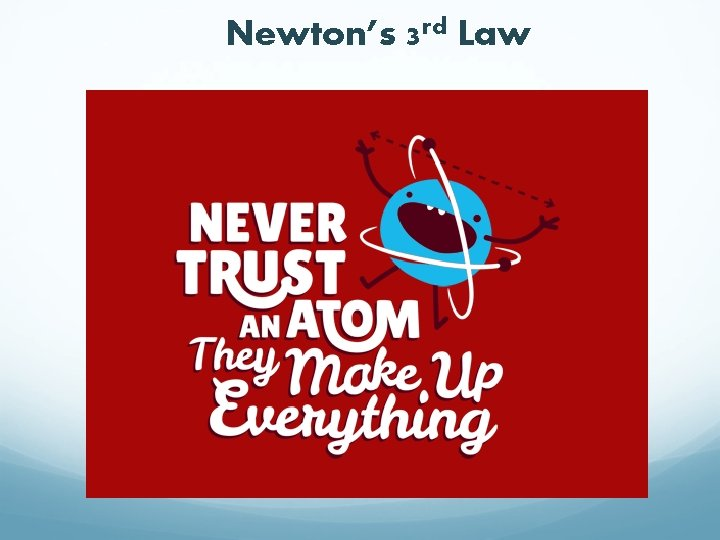Newton’s 3 rd LawNewton’s 3 rd Law Newton’s 2 nd Law is: A quantitative description of how forces affect motion. BUT: Where do forces come from? Answer this with EXPERIMENTS!! The results show that forces applied to an object are ALWAYS applied by another object Newton’s 3 rd Law: “Whenever one object exerts a force F on a second object, the second object exerts an equal and opposite force -F on the first object. ” Law of Action-Reaction: “Every action has an equal & opposite reaction”. (Note that action-reaction forces act on DIFFERENT objects!)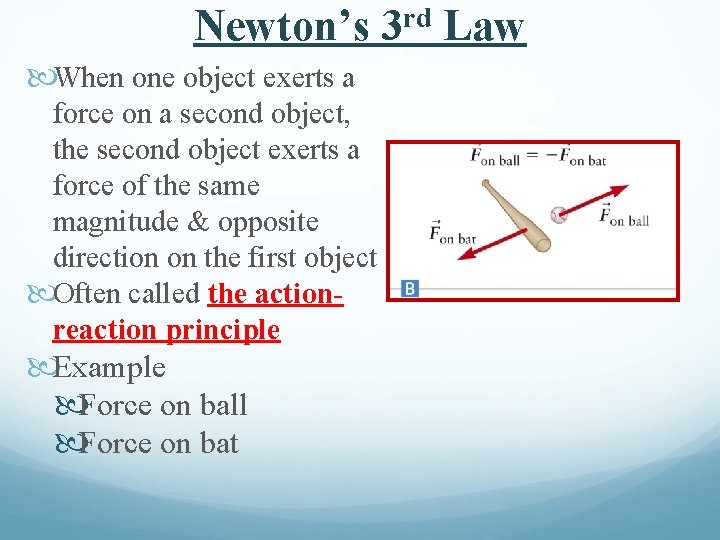Newton’s When one object exerts a force on a second object, the second object exerts a force of the same magnitude & opposite direction on the first object Often called the actionreaction principle Example Force on ball Force on bat rd 3 LawNewton’s Third Law Consequences Newton’s 3 rd Law tells us that FORCES COME IN PAIRS The 2 forces are always equal in magnitude & opposite in direction. IMPORTANT CONCEPT!! The 2 forces act on different objects Person exerts a force on the refrigerator The refrigerator exerts a force on the person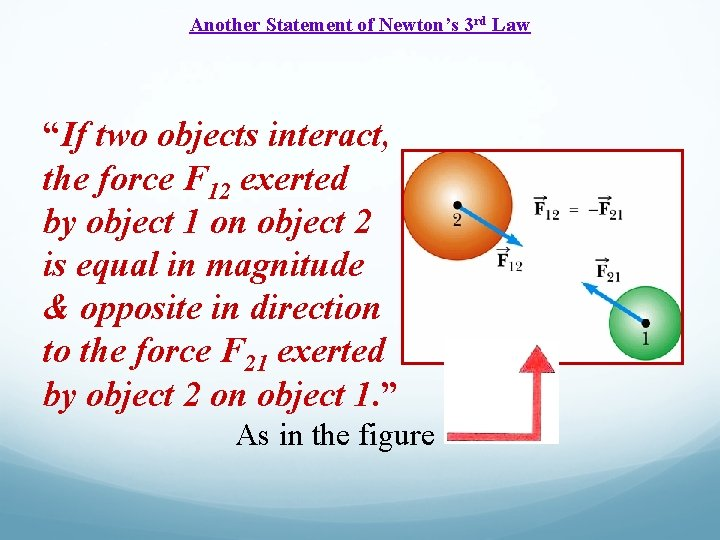Another Statement of Newton’s 3 rd Law “If two objects interact, the force F 12 exerted by object 1 on object 2 is equal in magnitude & opposite in direction to the force F 21 exerted by object 2 on object 1. ” As in the figureExample: Newton’s When a force is exerted on an object, that force is caused by another object. rd 3 Law Newton’s 3 rd Law: “Whenever one object exerts a force on a second object, the second exerts an equal force in the opposite direction on the first. ” If your hand pushes against the edge of a desk (red force vector), the desk pushes back against your hand (purple force vector; the two colors tell us that this force acts on a DIFFERENT object).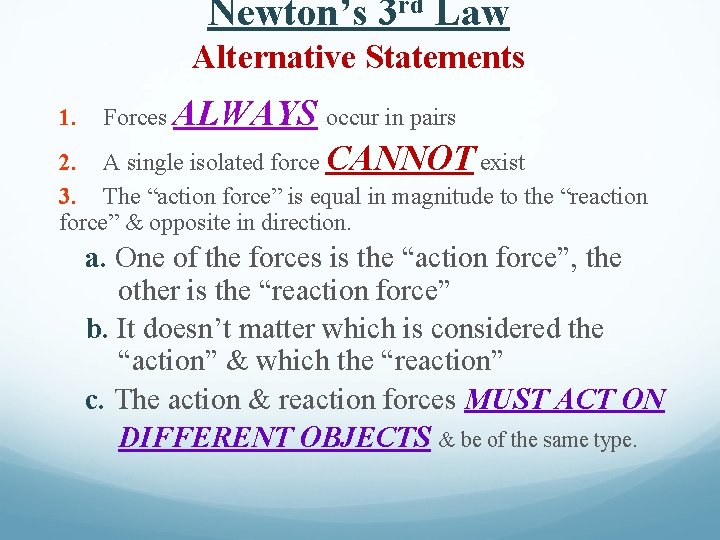Newton’s 3 rd Law Alternative Statements 1. Forces ALWAYS occur in pairs 2. A single isolated force CANNOT exist 3. The “action force” is equal in magnitude to the “reaction force” & opposite in direction. a. One of the forces is the “action force”, the other is the “reaction force” b. It doesn’t matter which is considered the “action” & which the “reaction” c. The action & reaction forces MUST ACT ON DIFFERENT OBJECTS & be of the same type.Action-Reaction Pairs: Act on Different Objects The key to correct application of Newton’s 3 rd Law is: THE FORCES ARE EXERTED ON DIFFERENT OBJECTS. Make sure you don’t use them as if they were acting on the same object. Example An ice skater pushes against a railing. The railing pushes back & this force causes her to move away.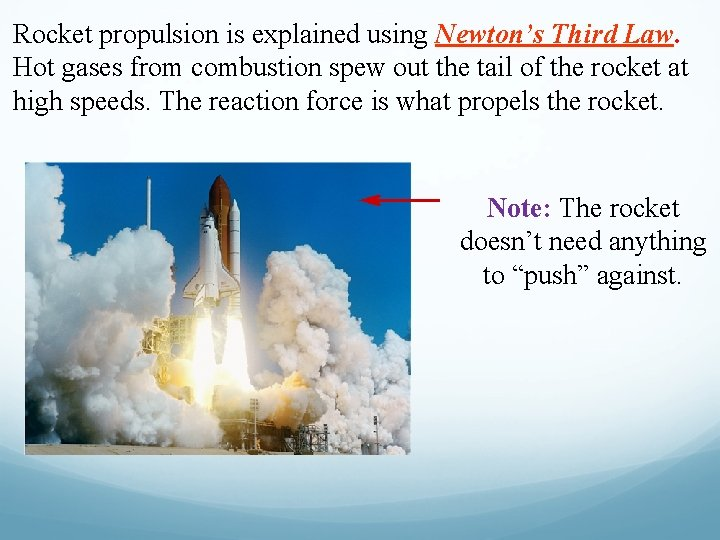Rocket propulsion is explained using Newton’s Third Law. Hot gases from combustion spew out the tail of the rocket at high speeds. The reaction force is what propels the rocket. Note: The rocket doesn’t need anything to “push” against.